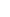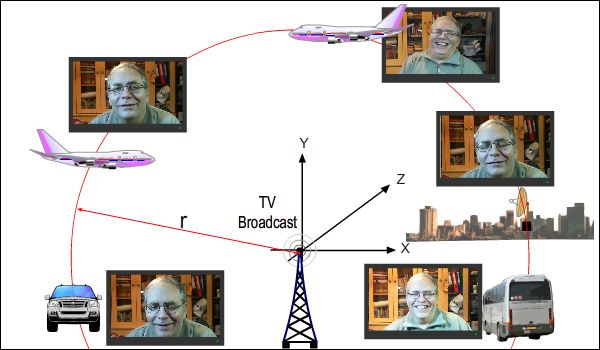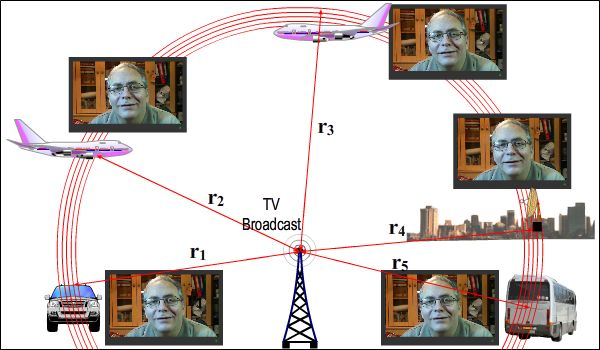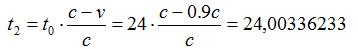24. SIMULTANEITYWe call it simultaneity when events happen at the same time. But how can we understand whether events happen at the same time or not? Is it simultaneous or is it not? We are going to give the answer to this question here.The information we got from the previous parts showed how we should deal with simultaneity. I wanted to collect all those bits and pieces of information under a single title.24.1. ABSOLUTE TIME AND SIMULTANEITYAs required by its nature, Absolute Time contains simultaneity within itself. For Alice Law, there is a principle decision indicating that a t moment that belongs to Absolute Time represents the same time value for all Source Objects in the universe. Therefore, if it cannot be disproved, there is no need to have even a shadow of doubt or hesitation. From this point of view, simultaneity is an easy recipe in Absolute Space-Time. If we say At the moment A and B masses collided, C and D masses collided, as well. in Absolute Space-Time, we will have explained what it is completely and accurately. However, the fact that Absolute Space-Time cannot be observed directly and that Source Objects cannot be seen prevents us from doing such explanations. If the real event takes place in Absolute Space-Time, simultaneity, in principle, must include a logic based on our understanding of whether events in Visible Space-Time occur in Absolute Space-Time at the same time. Space-Time at the same time.24.2. VISIBLE TIME AND SIMULTANEITYIn Visible Time, we dont have this simplicity and precision we have in Absolute Time. Just the opposite; there is huge chaos and confusion. No time unity between Image Objects in Visible Time, no location unity between Visible Coordinates, electromagnetic waves that carry the information on the event needing arrival time, and involvement of (c+v) (c-v) mathematics if there is movement make it extremely difficult to say an event occurs simultaneously. While an observer, based on his own observation, says At the moment A and B masses collided, C and D masses collided, as well., another observer may say that the collisions didnt happen at the same time for the same event.Simultaneity in Visible Space-Time is often deceptive. After all, Absolute Space-Time is the one to make the final decision whether the events are simultaneous or not.Reaching the correct information is only possible with the transition to Absolute Space-Time by using the information in Visible Space-Time. We saw them in an orderly fashion that this transition requires a period of analyses and calculations, (c+v) (c-v) mathematics can also be involved in when there are frames that are in motion relative to each other and also how to do the necessary calculations.24.3. SIMULTANEITY AND COEXISTENCE RULESOf course, there is not always a situation where we need to ask ourselves How did this happen in Absolute Space-Time? for all events we encounter. Id like to talk about how this situation is in practice. There are three important rules refer to whenever necessary in the topic of Simultaneity.Simultaneity Rules:1 - If observers that are moving at different speeds relative to a place of the incident are also at the same distance to the place of incident, each observer sees a different moment of the place of incident.2 - If observers that are moving at different speeds relative to a place of incident see the same moment of the event, the distance of each observer to the place of incident is different.3  Speed of flow of time of a place of incident is different for observers that are moving at different speeds relative to a place of incident.There are also Coexistence Rules, which are like siblings to Simultaneity Rules. We can call Coexistence Rules Equidistance Rules, as well.Coexistence (Equidistance) Rules1 - If observers that are moving at different speeds relative to a place of incident see that they are at the same distance to the place of incident, they are not at the same distance to the place of incident.2- If observers that are moving at different speeds relative to a place of incident are at the same distance to the place of incident, they see the place of incident at different distances.3 - For observers that are moving at different speeds relative to a place of incident, coordinates of the place of incident they see are always different from each other.All these rules explain and summarize the relationship between Absolute Space-Time and Visible Space-Time. As we saw the mathematical equations of these rules in parts before, I am not going to repeat them here.The figure above represents the first rule of Simultaneity. TV station (the place of incident) broadcasts an interview. There are reference systems that are moving at different speeds and directions relative to the TV station. If their distances to the TV station are the same for that moment, the image that each reference system sees is different from the others.The figure below represents the second rule. Lets think that reference systems are seeing the same image on their TVs. In this case, the distances of each reference system to the TV station are different.As I stated before, dont just think that these situations that we saw in the examples above take place on communication signals because electromagnetic waves that carry images of objects are, just like communication signals, subject to the rules of (c+v) (c-v) mathematics.Imagine we are watching a tennis match. Lets take the point we see the ball as the center and draw a circle of r radius in a way that it passes over us and other spectators. Each spectator on the circle sees a different t moment of the movement of the ball. In other words, when we saw the ball at a specific point, the ball didnt reach that point for some spectators; and others had seen the ball reaching that point before we did. It is not important how many nanoseconds or nanometers this difference is for the rule. As the distance and speed between reference systems increase, this difference becomes more evident. The important thing is that we are aware of these rules.By creating a problem, we can easily see some results of these rules in real life. Lets think that two space probes are moving at 150.000 km/h relative to the earth. Lets say that one is approaching the earth and the other is moving away from the earth. Assume that observers are watching a TV broadcast from the earth in both probes. We are discussing the situation when the probes are at the same distance to the earth. In order to have a 5 seconds difference between the broadcasts that observers watch, at what distance should the probes be from the earth?Answer: The difference between the arrival times of the TV signals to the probes should be 5 seconds. Based on this, we can find the distances of the probes from the earth.= 5.349.733.102 kilometters = 35,7841 AUc: speed of light: 299.792,458 km/sec, v: speed of probes = 150.000 km/h = 42 km/secWe found a huge distance as a result of the calculation. 35.78 AU is indeed a long distance; it is almost as much as the distance between Pluto and the Sun.
(AU: An astronomical unit. It is based on the distance between the center of the sun and the center of the earth and it corresponds to 149.5 million kilometers.)Lets keep working on the same example with a second question. How far away do the observers see the earth from themselves at that moment?Answer: Now that we calculated the distances of the probes to the earth, we can calculate the distances at which the observers in the probes see the earth.For the probe that is approaching the earth.= 5.350.482.688 kilometers = 35,7892 AU
For the probe that is moving away from the earth:= 5.348.983.726 kilometers = 35,7792 AULets ask a more interesting question now. What is the duration of rotation of the earth around its own axis for the observers in the probes?Answer: The earth completes its rotation around its own axis in 24 hours. We can calculate this as we know the speeds of the probes.The duration of rotation of the earth around its own axis relative to the probe that is moving away:23,99663767 hours = 23 hours 59 minutes 47 secondsThe duration of rotation of the earth around its own axis relative to the probe that is approaching:24,00336233 hours = 24 hours 0 minutes 10 secondsThe speed value 150.000 km/h that we chose in the questions above is hard to reach even todays world. Nevertheless, we see that there are no serious differences in terms of Relativity Effects. For these effects to be more obvious, we need to have a lot higher speeds. The table below has been prepared based on 0.1c  0.9c values for the speeds of the probes, but for the situation where 1 second instead of 5 seconds of the broadcast shift. The questions are the same and the answers are in the table. In the table, you can see how the values go crazy as the speed goes up.These were the questions:
Both probes are at the same distance from the earth.
1- In order to have 1-second difference between the broadcasts that observers watch, at what distance should the probes be from the earth?
2- How far away do the observers see the earth from themselves at that moment?
3- What is the duration of rotation of the earth around its own axis for the observers in the probes?The answers are in the table: